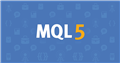# returns the execution policy.

`long  SymbolInfoInteger(_Symbol,SYMBOL_FILLING_MODE);`
returned values

iok is 2
fok is 1
gtc is 0

Is this correct? Can't there be two policies available?

Ivan_Invanov:
returned values

iok is 2
fok is 1
gtc is 0

Is this correct? Can't there be two policies available?

There can be one and two ...

```//+------------------------------------------------------------------+
//| проверяет разрешенность указанного режима заполнения             |
//+------------------------------------------------------------------+
bool IsFillingTypeAllowed(string symbol,int fill_type)
{
//--- получим значение свойства, описывающего режим заполнения
int filling=(int)SymbolInfoInteger(symbol,SYMBOL_FILLING_MODE);
//--- вернем true, если режим fill_type разрешен
return((filling&fill_type)==fill_type);
}```Документация по MQL5: Константы, перечисления и структуры / Состояние окружения / Информация об инструменте
• www.mql5.com
Для получения текущей рыночной информации служат функции SymbolInfoInteger(), SymbolInfoDouble() и SymbolInfoString(). В качестве второго параметра этих функций допустимо передавать один из идентификаторов из перечислений ENUM_SYMBOL_INFO_INTEGER, ENUM_SYMBOL_INFO_DOUBLE и ENUM_SYMBOL_INFO_STRING соответственно. Некоторые символы (как правило...

a bit check is needed. for example:

```ENUM_ORDER_TYPE_FILLING OrderTypeFilling(const string symbol)
{
int FillingFlags = (int)SymbolInfoInteger(symbol, SYMBOL_FILLING_MODE);

if((FillingFlags & SYMBOL_FILLING_FOK) == SYMBOL_FILLING_FOK)
return ORDER_FILLING_FOK;
else
if((FillingFlags & SYMBOL_FILLING_IOC) == SYMBOL_FILLING_IOC)
return ORDER_FILLING_IOC;
else
return ORDER_FILLING_RETURN;
}```

There may be one or two ...

Thank you. Can you also explain why they sometimes write break in the if statement, an example from the official advisor.
```if(x>0.0)
break;
if(x<0.0)
y++;
```

Why is this not the same as return. Return will return control to the calling program, which proceeds to the next operator. Break forces a transition to the next operator. Can the calling program ignore the next operator?

Ivan_Invanov:
Thank you. Can you also explain why break is sometimes written in if statement, example from official advisor.

Why is this not the same as return. Return will return control to the calling program, which proceeds to the next statement. break forces the program to go to the next operator. Can the calling program ignore the next operator?

Give me a link to the documentation where this

```if(x>0.0)
break;
if(x<0.0)
y++;```

example ...

This is from the Moving Average v1.00 2009-2017 .It is an example in the mt5 terminal. Line 64.

Ivan_Invanov:
This is from Moving Average v1.00 2009-2017 .It's an example in the mt5 terminal. Line 64.

1. Don't try to take the code out of context.

2. Read the End Break Operator Help carefully.

3. Only after you have completed tasks 1 and 2 can you continue to ask questions.Документация по MQL5: Основы языка / Операторы / Оператор завершения break
• www.mql5.com
Основы языка / Операторы / Оператор завершения break - справочник по языку алгоритмического/автоматического трейдинга для MetaTrader 5

1. Don't try to take the code out of context.

2. Read the End Break Operator Help carefully.

3. Only after you have completed tasks 1 and 2 can you continue to ask questions.

Thank you. I'm deleting the thread so as not to clutter up the forum. Ah, you're not allowed to delete threads here.

There may be one or two ...

I don't understand, you're saying it can be one or two. There is only one variable in the function. Can you tell me with an example.

Ivan_Invanov:
I don't understand, you're saying it could be one or two. There is only one variable in the function. Can you tell me with an example.

iok is 2
fok is 1
gtc is 0

what then returns if ioc and fok

Ivan_Invanov:
I don't understand, you are saying that there can be one or two. There is only one variable in the function. Can you tell me with an example.

Example from CTrade trade class. First it is checked for'SYMBOL_FILLING_FOK', then for'SYMBOL_FILLING_IOC'.

```//+------------------------------------------------------------------+
//| Set order filling type according to symbol filling mode          |
//+------------------------------------------------------------------+
{
//--- get possible filling policy types by symbol
uint filling=(uint)SymbolInfoInteger(symbol,SYMBOL_FILLING_MODE);
if((filling&SYMBOL_FILLING_FOK)==SYMBOL_FILLING_FOK)
{
m_type_filling=ORDER_FILLING_FOK;
return(true);
}
if((filling&SYMBOL_FILLING_IOC)==SYMBOL_FILLING_IOC)
{
m_type_filling=ORDER_FILLING_IOC;
return(true);
}
//---
return(false);
}```

The check is performed by usingthe 'AND bitwise operation'.

### Bitwise AND operation

The bitwise AND operation of the binary representations x and y. The value of the expression contains 1 (TRUE) in all bits where both x and y contain non-zero; and 0 (FALSE) in all other bits.

`b = ((x & y) != 0);`

Example:

```   char a='a',b='b';
//--- операция И
char c=a&b;
Print("a = ",a,"  b = ",b);
Print("a & b = ",c);
// Результат будет такой:
// a = 97   b = 98
// a & b = 96```